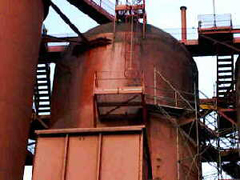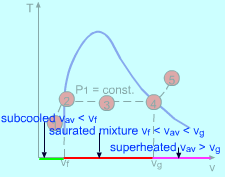Ch 2. Pure Substances Multimedia Engineering Thermodynamics Phase PropertyDiagrams PropertyTables IdealGas
 Chapter 1. Basics 2. Pure Substances 3. First Law 4. Energy Analysis 5. Second Law 6. Entropy 7. Exergy Analysis 8. Gas Power Cyc 9. Brayton Cycle 10. Rankine Cycle Appendix Basic Math Units Thermo Tables eBooks Dynamics Fluids Math Mechanics Statics Thermodynamics Author(s): Meirong Huang Kurt Gramoll ©Kurt GramollTHERMODYNAMICS - CASE STUDY IntroductionBoiler in the Power Plant John got a new job in a power plant. His job is to control a boiler that is used to produce steam and which will be sent to the turbine for power generation. The only property that can be read directly from the monitor provided is the pressure. In order to monitor the vaporization process, he needs to know the temperature of the water and how much liquid water left at some pressure. What is known: The pressure in the boiler is 5 MPa The volume of the boiler is 10 m3 and the mass of water is 500 kg QuestionsSteps to Determine the States of Water Determine the temperature of water in the boiler Determine the total enthalpy Determine the mass of each phase of water Approach Consider water in the boiler as a closed system Use the specific volume and pressure to locate the state on the P-v diagram